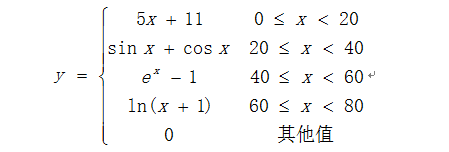#include"stdio.h"
#include"math.h"
void main()
{
float x;
float y;
printf("input x:");
scanf("%f",&x);
(0<=x&&x y=sin(x)+cos(x):
(40 y=exp(x)-1:
(60 y=log(x+1):
(0>x&&x>=80);
printf("y=0");
printf("%f",y);
}

8个回答

`````` #include "stdio.h"
#include "math.h"
int main()
{
float x;
float y;
printf("input x:");
scanf("%f",&x);
y=(0<=x&&x<20)?5*x+11:
(
(20<=x&&x<40)?sin(x)+cos(x):
(
(40<=x&&x<60)?exp(x)-1:
(
(60<=x&&x<80)?log(x+1):0
)
)
);
printf("%f",y);
}
``````

if else if ... else 比较直观，不要用条件运算符。

`````` #include <math.h>

int main()
{
double x = 0, y=0;
printf("请输入x的值");
scanf("%lf", &x);
if (x >= 0 && x < 20) {
y = 5 * x + 11;
}
else if (x >= 20 && x < 40) {
y = sin(x) + cos(x);
}
else if (x >= 40 && x < 60) {
y = exp(x) - 1;
}
else if (x >= 60 && x < 80) {
y = log(x + 1);
}
else {
y = 0;
}
printf("%f", y);
return 0;
}
``````

#include"stdio.h"
#include"math.h"
void main()
{
float x;
float y;
printf("input x:");
scanf("%f",&x);
(0<=x&&x y=sin(x)+cos(x):
(40 y=exp(x)-1:
(60 y=log(x+1):
(0>x&&x>=80);
printf("y=0");
printf("%f",y);
}